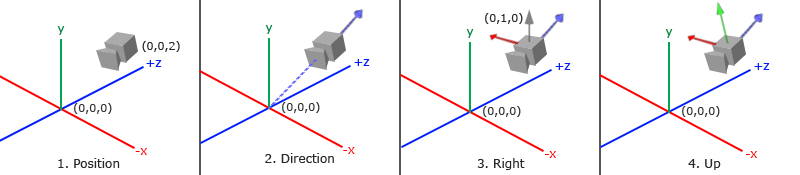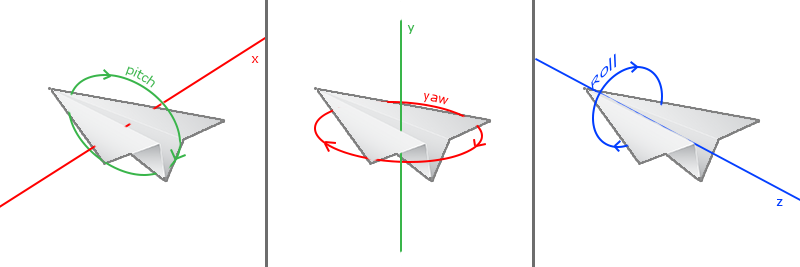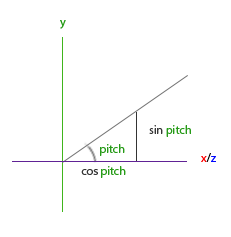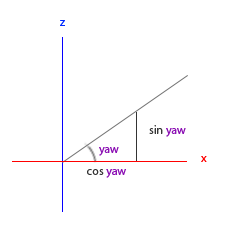# 摄像机(Camera)

## 摄像机/观察空间### 1. 摄像机位置

glm::vec3 cameraPos = glm::vec3(0.0f, 0.0f, 3.0f);


Important

### 2. 摄像机方向

glm::vec3 cameraTarget = glm::vec3(0.0f, 0.0f, 0.0f);
glm::vec3 cameraDirection = glm::normalize(cameraPos - cameraTarget);


Attention

### 3. 右轴

glm::vec3 up = glm::vec3(0.0f, 1.0f, 0.0f);
glm::vec3 cameraRight = glm::normalize(glm::cross(up, cameraDirection));


#### 4. 上轴

glm::vec3 cameraUp = glm::cross(cameraDirection, cameraRight);


## Look At

$\color{red}R$是右向量，$\color{green}U$是上向量，$\color{blue}D$是方向向量$\color{purple}P$是摄像机位置向量。注意，位置向量是相反的，因为我们最终希望把世界平移到与我们自身移动的相反方向。使用这个LookAt矩阵坐标观察矩阵可以很高效地把所有世界坐标变换为观察坐标LookAt矩阵就像它的名字表达的那样：它会创建一个观察矩阵looks at(看着)一个给定目标。

glm::mat4 view;
view = glm::lookAt(glm::vec3(0.0f, 0.0f, 3.0f),
glm::vec3(0.0f, 0.0f, 0.0f),
glm::vec3(0.0f, 1.0f, 0.0f));


glm::LookAt函数需要一个位置、目标和上向量。它可以创建一个和前面所说的同样的观察矩阵。

GLfloat radius = 10.0f;
GLfloat camX = sin(glfwGetTime()) * radius;
GLfloat camZ = cos(glfwGetTime()) * radius;
glm::mat4 view;
view = glm::lookAt(glm::vec3(camX, 0.0, camZ), glm::vec3(0.0, 0.0, 0.0), glm::vec3(0.0, 1.0, 0.0));


# 自由移动

glm::vec3 cameraPos   = glm::vec3(0.0f, 0.0f,  3.0f);
glm::vec3 cameraFront = glm::vec3(0.0f, 0.0f, -1.0f);
glm::vec3 cameraUp    = glm::vec3(0.0f, 1.0f,  0.0f);


LookAt函数现在成了：

view = glm::lookAt(cameraPos, cameraPos + cameraFront, cameraUp);


void key_callback(GLFWwindow* window, int key, int scancode, int action, int mode)
{
...
GLfloat cameraSpeed = 0.05f;
if(key == GLFW_KEY_W)
cameraPos += cameraSpeed * cameraFront;
if(key == GLFW_KEY_S)
cameraPos -= cameraSpeed * cameraFront;
if(key == GLFW_KEY_A)
cameraPos -= glm::normalize(glm::cross(cameraFront, cameraUp)) * cameraSpeed;
if(key == GLFW_KEY_D)
cameraPos += glm::normalize(glm::cross(cameraFront, cameraUp)) * cameraSpeed;
}


Important

bool keys;


if(action == GLFW_PRESS)
keys[key] = true;
else if(action == GLFW_RELEASE)
keys[key] = false;


void do_movement()
{
// 摄像机控制
GLfloat cameraSpeed = 0.01f;
if(keys[GLFW_KEY_W])
cameraPos += cameraSpeed * cameraFront;
if(keys[GLFW_KEY_S])
cameraPos -= cameraSpeed * cameraFront;
if(keys[GLFW_KEY_A])
cameraPos -= glm::normalize(glm::cross(cameraFront, cameraUp)) * cameraSpeed;
if(keys[GLFW_KEY_D])
cameraPos += glm::normalize(glm::cross(cameraFront, cameraUp)) * cameraSpeed;
}


while(!glfwWindowShouldClose(window))
{
// 检测并调用事件
glfwPollEvents();
do_movement();

// 渲染
...
}


## 移动速度

GLfloat deltaTime = 0.0f;   // 当前帧遇上一帧的时间差
GLfloat lastFrame = 0.0f;   // 上一帧的时间


GLfloat currentFrame = glfwGetTime();
deltaTime = currentFrame - lastFrame;
lastFrame = currentFrame;


void Do_Movement()
{
GLfloat cameraSpeed = 5.0f * deltaTime;
...
}


# 视角移动

## 欧拉角direction.y = sin(glm::radians(pitch)); // 注意我们先把角度转为弧度


direction.x = cos(glm::radians(pitch));direction.x = cos(glm::radians(pitch)) * cos(glm::radians(yaw));//译注：direction代表摄像机的“前”轴，但此前轴是和本文第一幅图片的第二个摄像机的direction是相反的


## 鼠标输入

glfwSetInputMode(window, GLFW_CURSOR, GLFW_CURSOR_DISABLED);


void mouse_callback(GLFWwindow* window, double xpos, double ypos);


glfwSetCursorPosCallback(window, mouse_callback);


1. 计算鼠标和上一帧的偏移量。
2. 把偏移量添加到摄像机和俯仰角和偏航角中。
3. 对偏航角和俯仰角进行最大和最小值的限制。
4. 计算方向向量。

GLfloat lastX = 400, lastY = 300;


GLfloat xoffset = xpos - lastX;
GLfloat yoffset = lastY - ypos; // 注意这里是相反的，因为y坐标的范围是从下往上的
lastX = xpos;
lastY = ypos;

GLfloat sensitivity = 0.05f;
xoffset *= sensitivity;
yoffset *= sensitivity;


yaw   += xoffset;
pitch += yoffset;


if(pitch > 89.0f)
pitch =  89.0f;
if(pitch < -89.0f)
pitch = -89.0f;


glm::vec3 front;
cameraFront = glm::normalize(front);


if(firstMouse) // 这个bool变量一开始是设定为true的
{
lastX = xpos;
lastY = ypos;
firstMouse = false;
}


void mouse_callback(GLFWwindow* window, double xpos, double ypos)
{
if(firstMouse)
{
lastX = xpos;
lastY = ypos;
firstMouse = false;
}

GLfloat xoffset = xpos - lastX;
GLfloat yoffset = lastY - ypos;
lastX = xpos;
lastY = ypos;

GLfloat sensitivity = 0.05;
xoffset *= sensitivity;
yoffset *= sensitivity;

yaw   += xoffset;
pitch += yoffset;

if(pitch > 89.0f)
pitch = 89.0f;
if(pitch < -89.0f)
pitch = -89.0f;

glm::vec3 front;
cameraFront = glm::normalize(front);
}


## 缩放

void scroll_callback(GLFWwindow* window, double xoffset, double yoffset)
{
if(aspect >= 1.0f && aspect <= 45.0f)
aspect -= yoffset;
if(aspect <= 1.0f)
aspect = 1.0f;
if(aspect >= 45.0f)
aspect = 45.0f;
}


yoffset值代表我们滚动的大小。当scroll_callback函数调用后，我们改变全局aspect变量的内容。因为45.0f是默认的fov，我们将会把缩放级别限制在1.0f45.0f

projection = glm::perspective(aspect, (GLfloat)WIDTH/(GLfloat)HEIGHT, 0.1f, 100.0f);


glfwSetScrollCallback(window, scroll_callback);


Important

# 摄像机类

Attention

## 练习

• 看看你是否能够变换摄像机类从而使得其能够变- 成一个真正的FPS摄像机(也就是说不能够随意飞行)；你只能够呆在xz平面上: 参考解答

• 试着创建你自己的LookAt函数，使你能够手动创建一个我们在一开始讨论的观察矩阵。用你的函数实现来替换glm的LookAt函数，看看它是否还能一样的工作：参考解答